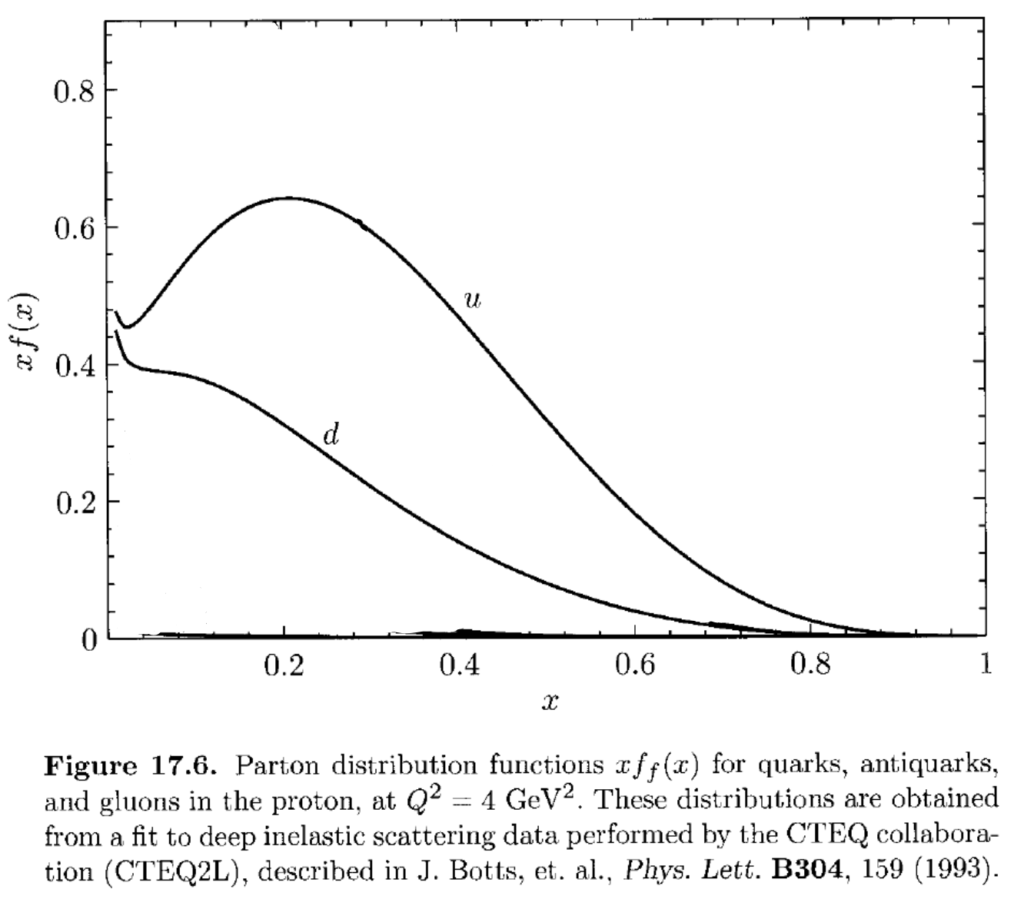# Proton Momentum: Hiding in Plain Sight?

Protons and neutrons at first glance seem like simple objects. They have well defined spin and electric charge, and we even know their quark compositions. Protons are composed of two up quarks and one down quark and for neutrons, two downs and one up. Further, if a proton is moving, it carries momentum, but how is this momentum distributed between its constituent quarks? In this post, we will see that most of the momentum of the proton is in fact not carried by its constituent quarks.

Before we start, we need to have a small discussion about isospin. This will let us immediately write down the results we need later. Isospin is a quantum number that in practice, allows us to package particles together. Protons and neutrons form an isospin doublet, which means they come in the same mathematical package. The proton is the isospin +1/2 component of this package, and the neutron is the isospin -1/2 component of this package. Similarly, up quarks and down quarks form their own isospin doublet, and they come in their own package. In our experiment, if we are careful to choose which particles to scatter off of eachother, our calculations will permit us to exchange components of isospin packages everywhere instead of redoing calculations from scratch. This exchange is what I will call the “isospin trick.” It turns out that if compare electron-proton scattering to electron-neutron scattering allows us to use this trick:$\text{Proton} \leftrightarrow \text{Neutron} \\ u \leftrightarrow d$

Back to protons and neutrons. We know that protons and neutrons are composite particles, they themselves are made up of more fundamental objects. We need a way to “zoom into” these composite particles, to look inside them and we do this with the help of structure functions$F(x)$. Structure functions for the proton and neutron encode how electric charge and momentum are distributed between the constituents. We assign$u(x)$ and$d(x)$ to be the probability of finding an up or down quark with momentum fraction$x$ of the proton. Explicitly, these structure functions look like:$F(x) \equiv \sum_q e_q^2 q(x) \\ \ F_P(x) = \frac{4}{9}u(x) + \frac{1}{9}d(x) \\ \ F_N(x) = \frac{4}{9}d(x) + \frac{1}{9}u(x)$

where the first line is the definition of a structure function. In this line,$q$ denotes quarks, and$e_q$ is the electric charge of quark$q$. In the second line, we have written out explicitly the structure function for the proton$F_P(x)$, and invoked the isospin trick to immediately write down the structure function for the neutron$F_N(x)$ in the third line. Observe that if we had attempted to write down$F_N(x)$ following the definition in line 1, we would have gotten the same thing as the proton.

At this point we must turn to experiment to determine$u(x)$ and$d(x)$. The plot we will examine  is figure 17.6 taken from section 17.4 of Peskin and Schroeder, An Introduction to Quantum Field Theory. Some data is omitted to illustrate a point.The momentum distribution of the quarks inside the proton. Some data has been omitted for the purposes of this discussion. The full plot is provided at the end of this post.

This plot shows the momentum distribution of the up and down quarks inside a proton. On the horizontal axis is the momentum fraction$x$ and on the vertical axis is probability. The two curves represent the probability distribution of the up (u) and down (d) quarks inside the proton. Integrating these curves gives us the total percent of momentum stored in the up and down quarks which I will call uppercase$U$ and uppercase$D$. We want to know know both$U$ and$D$, so we need another equation to solve this system. Luckily we can repeat this experiment using neutrons instead of protons, obtain a similar set of curves, and integrate them to obtain the following system of equations:$\int_0^1 F_P(x) = \frac{4}{9}U(x) + \frac{1}{9}D(x) = 0.18 \\ \int_0^1 F_N(x) = \frac{4}{9}D(x) + \frac{1}{9}U(x) = 0.12$

Solving this system for$U$ and$D$ yields$U = 0.36$ and$D = 0.18$. We immediately see that the total momentum carried by the up and down quarks is$\sim 54%$ of the momentum of the proton. Said a different way, the three quarks that make up the proton, only carry half ot its momentum. One possible conclusion is that the proton has more “stuff” inside of it that is storing the remaining momentum. It turns out that this additional “stuff” are gluons, the mediators of the strong force. If we include gluons (and anti-quarks) in the momentum distribution, we can see that at low momentum fraction$x$, most of the proton momentum is stored in gluons. Throughout this discussion, we have neglected anti-quarks because even at low momentum fractions, they are sub-dominant to gluons. The full plot as seen in Peskin and Schroeder is provided below for completeness.References

 – Peskin and Schroeder, An Introduction to Quantum Field Theory, Section 17.4, figure 17.6.

[A] – The uses of isospin in early nuclear and particle physics. This article follows the historical development of isospin and does a good job of motivating why physicists used it in the first place.

[B] – Fundamentals in Nuclear Theory, Ch3. This is a more technical treatment of isospin, roughly at the level of undergraduate advanced quantum mechanics.

[C] – Symmetries in Physics: Isospin and the Eightfold Way. This provides a slightly more group-theoretic perspective of isospin and connects it to the SU(3) symmetry group.

[D] – Structure Functions. This is the Particle Data Group treatment of structure functions.

[E] – Introduction to Parton Distribution Functions]. Not a paper, but an excellent overview of Parton Distribution Functions.

The following two tabs change content below.